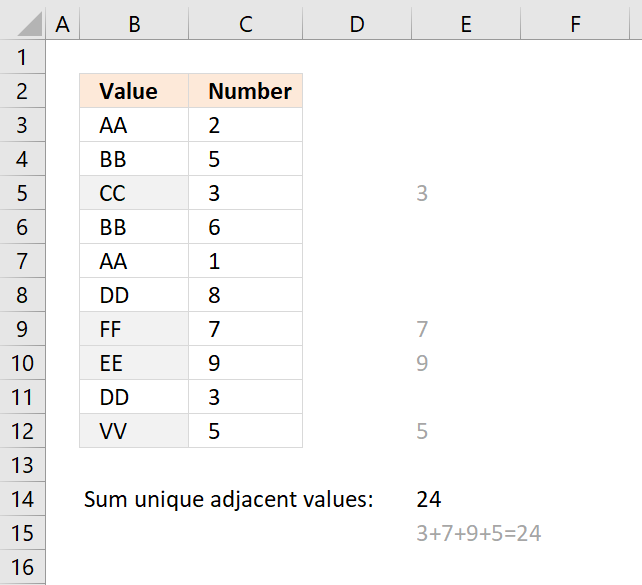Author: Oscar Cronquist Article last updated on December 21, 2018The formula in cell E14 adds a number from column C if the corresponding value in column B is unique and returns the total for all unique values. Unique values are values that exist only once in the list, in other words, there is only one instance of the value.

Formula in cell E14:

=SUMPRODUCT((COUNTIF(\$B\$3:\$B\$12,\$B\$3:\$B\$12)=1)*\$C\$3:\$C\$12)

### Explaining formula in cell E14

#### Step 1 - Count values in column B

The COUNTIF function counts values based on a condition, I am using multiple conditions, in this case.

COUNTIF(\$B\$3:\$B\$12,\$B\$3:\$B\$12)

becomes

COUNTIF({"AA";"BB";"CC";"BB";"AA";"DD";"FF";"EE";"DD";"VV"}, {"AA";"BB";"CC";"BB";"AA";"DD";"FF";"EE";"DD";"VV"})

and returns

{2;2;1;2;2;2;1;1;2;1}

#### Step 2 - Check if unique

The equal sign compares each value in the array to 1 and returns either TRUE or FALSE.

COUNTIF(\$B\$3:\$B\$12,\$B\$3:\$B\$12)=1

becomes

{2;2;1;2;2;2;1;1;2;1}=1

and returns

{FALSE; FALSE; TRUE; FALSE; FALSE; FALSE; TRUE; TRUE; FALSE; TRUE}

#### Step 3 - Multiply with numbers in column C

(COUNTIF(\$B\$3:\$B\$12,\$B\$3:\$B\$12)=1)*\$C\$3:\$C\$12

becomes

{FALSE; FALSE; TRUE; FALSE; FALSE; FALSE; TRUE; TRUE; FALSE; TRUE}*\$C\$3:\$C\$12

becomes

{FALSE; FALSE; TRUE; FALSE; FALSE; FALSE; TRUE; TRUE; FALSE; TRUE}*{2;5;3;6;1;8;7;9;3;5}

and returns

{0;0;3;0;0;0;7;9;0;5}

#### Step 4 - SUM numbers

The SUMPRODUCT function then adds the numbers and returns the total to cell E14.

SUMPRODUCT((COUNTIF(\$B\$3:\$B\$12,\$B\$3:\$B\$12)=1)*\$C\$3:\$C\$12)

becomes

SUMPRODUCT({0;0;3;0;0;0;7;9;0;5})

and returns

24 in cell E14.